## Tuesday, October 15, 2013

### Calculus Lesson: Area Between Curves

Area Between Two Curves

The general formula for finding the area between two curves is:

b
∫ T(x) - B(x) dx
a

I named the functions T(x) and B(x) specifically. T(x) represents the function "on top", while B(x) represents the function "on the bottom". In short, for x ∈ (a,b), T(x) ≥ B(x).

Here is an approach to use when finding areas between curves. A graphing calculator or mathematical software can be helpful in this procedure.

1. Draw the graphs. This is where a graphing calculator comes in handy.

2. Determine a and b, your limit points. Sometimes one or both of the limit points are found by finding the intersection of the two curves. Other problems will give you a third (or even fourth) condition. An example is x≥0, which makes one of our limit points x=0.

3. Determine which of the curves is T(x) and B(x). T(x) has greater numerical value than B(x) over the interval x ∈ (a, b).

4. Take the definite integral of T(x) - B(x). The result is your area.

b
∫ T(x) - B(x) dx = Area
a

About the examples and problem set: Please note that most examples in math textbooks, the numbers come out "nice and neat". Not so here, so I am using an HP Prime to demonstrate these examples and come up with answers. (any graphing calculator, HP, TI, Casio, all will do just fine!)

Example 1:

Find the area between:
y = -x^2 + 2
y = x^2 + 1

Here is a graph of the two curves: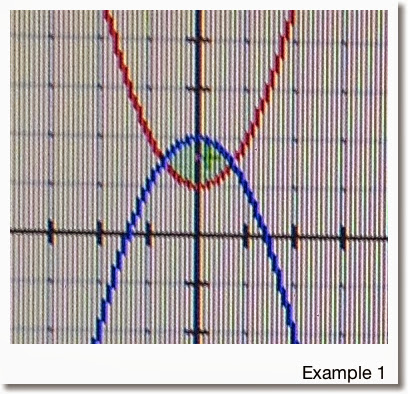Finding the limit points:

-x^2 + 2 = x^2 + 1
1 = 2*x^2
1/2 = x^2
Which implies x = -1/√2 and x = 1/√2

So our limit points are a = -1/√2 and b = 1/√2

From the graph we note that:
T(x) = -x^2 + 2
B(x) = x^2 + 1
and
T(x) - B(x) = -x^2 + 2 - (x^2 + 1) = -2*x^2 + 1

Taking the integral:

1/√2
∫ -2*x^2 + 1 dx ≈ 0.94281
-1/√2

So the area for Example 1 is about 0.94281.

Example 2:

Find the area between the two curves:
y = 2 cos x
y = x - 2
With the condition x≥0

First we graph the two curves: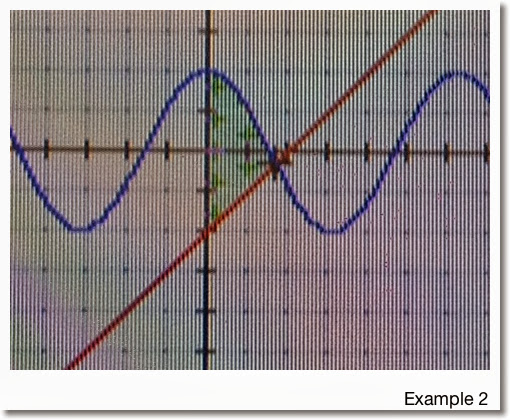The two graphs intersect when x>0. Since we are given the additional condition that x≥0, the lower limit for this problem is a=0.

To find the upper limit, we find intersection point:
2 cos x = x - 2
2 cos x - x + 2 = 0

Using a numeric solver on a calculator, we find that the intersection point is x ≈ 1.71419. (See, the numbers in these examples aren't always as "neat").

In the region x ∈ (0, 1.71419), we see that 2 cos x > x - 2. Hence:

T(x) = 2 cos x
B(x) = x - 2
and the area is approximately

1.71419
∫ 2 cos x - (x + 2) dx ≈ 3.93863
0

Problems to Try

Problem 1
Find the area between the curves of
y = x^2
y = 4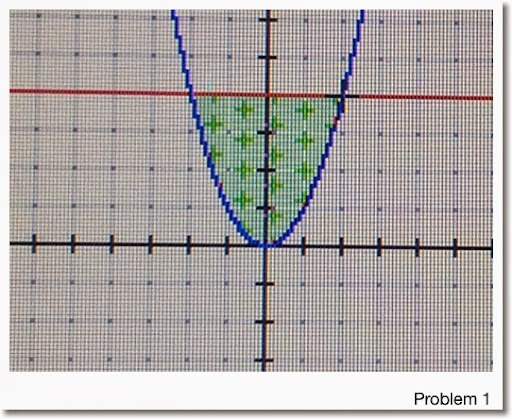Problem 2

y = x^3
y = -3*x + 8
With the condition x≥0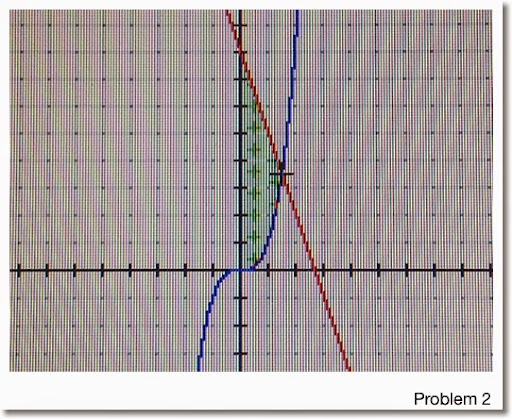Problem 3

y = 8*x^2 + 4*x - 6
y = x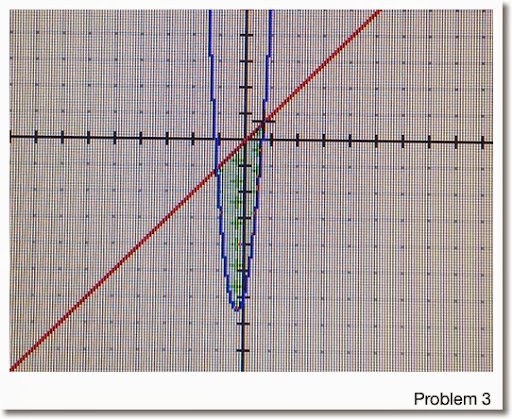Areas are rounded to five decimal points:
1. 10.66667
2. 7.36017 (Hint: Upper Limit ≈ 1.51275)
3. 7.42101 (Hint: Use a calculator to find the limit points)

Any questions, please leave them in the comment box. Hope this is helpful and thanks!

Eddie

This blog is property of Edward Shore. 2013

1.This comment has been removed by the author.

2.1.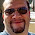Jonathan,

3.